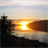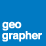# variable in modelbuilder?

1880
1
06-19-2014 01:22 AMby
New Contributor
Hello everyone!

I got a problem in modelbuilder. I need use "select by attribute" function to pick up some values that are less than the mean value. However, I dont know how can I define or input this mean value.

To be specific, I have a polyline shapfile and with a length field. Then I calculate its mean value (Length field) by right clicking fields - stasticis. here,I got mean value and type it as a input in "select by attribute" function by:

Length< mean (for example 1000)  Length <1000

Then, Arcgis can select  lengths that are less than mean value (1000). Then I used  "Feature to polygon" function to output this features. Please note this is a manual way.

In modelbuilder, I have to drag some functional tools to make this possible. The problem was that the result calculated by "summary stastics" function only output a new table file, and this file was not related with previous work. My question is how ArcGIS can understand if I would like to use mean value (located in new table fild) as a threshold to select the length. In other words, How this mean value can be defined as a variable and identified when working with "Select by attribute" function (actually, there was only one number useful, not the whole table).

My supposed result like this: calculate mean value - select lengths (according to the mean value and less than this value)- feature to polygon as output.

I don't have any background in PC language and SQL, but I think it could work.
For example:
1. calculate mean value
2. Define mean value as a variable
3. Select by attribute : e.g length < mean?
...

I think there will be some SQL language or code in play but dont know what exactly I can do for this process. The reason why I would like to make this possible is that I have a big project so that making a fast and complete model for whole project would be very helpful.

I hope if someone can kindly help me or give me some tips.
I would be much appreciate.
Tags (3)